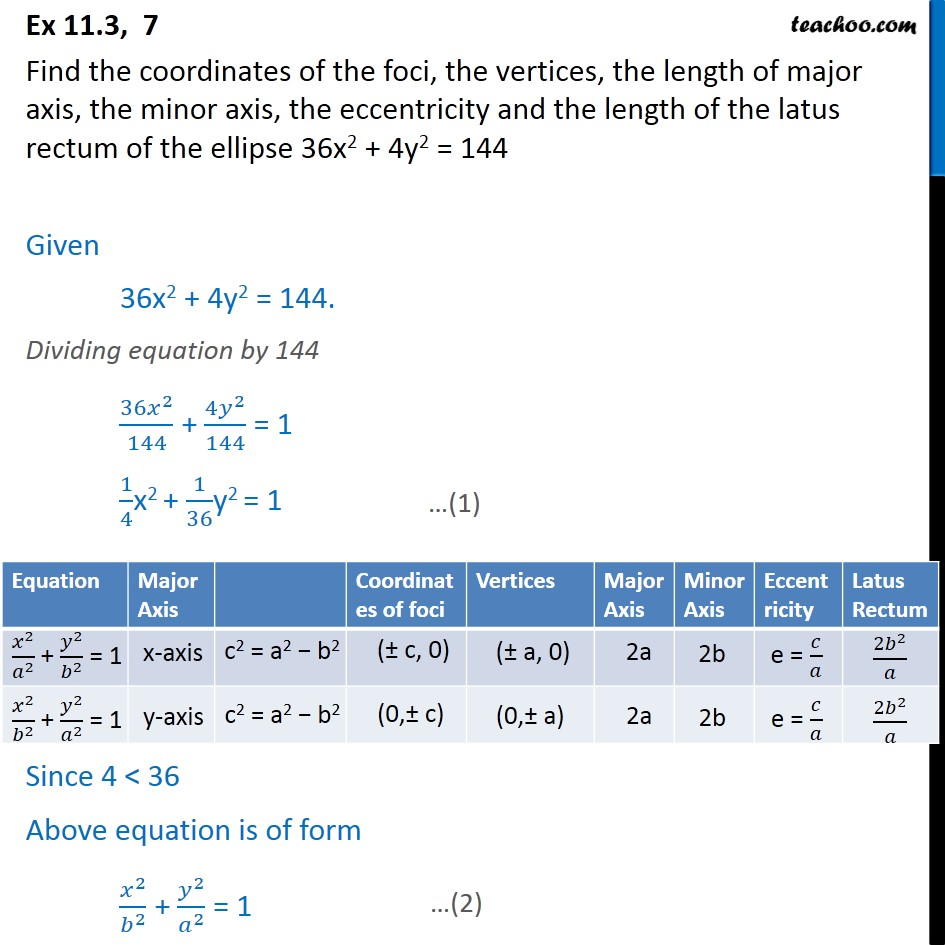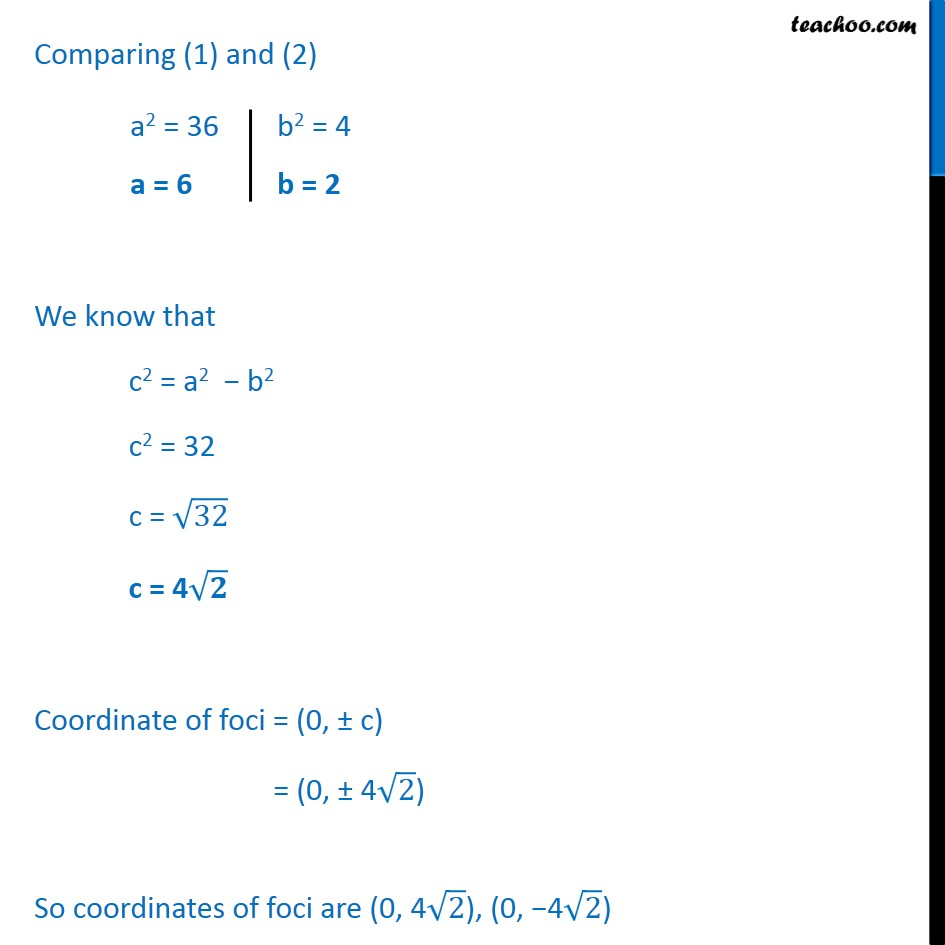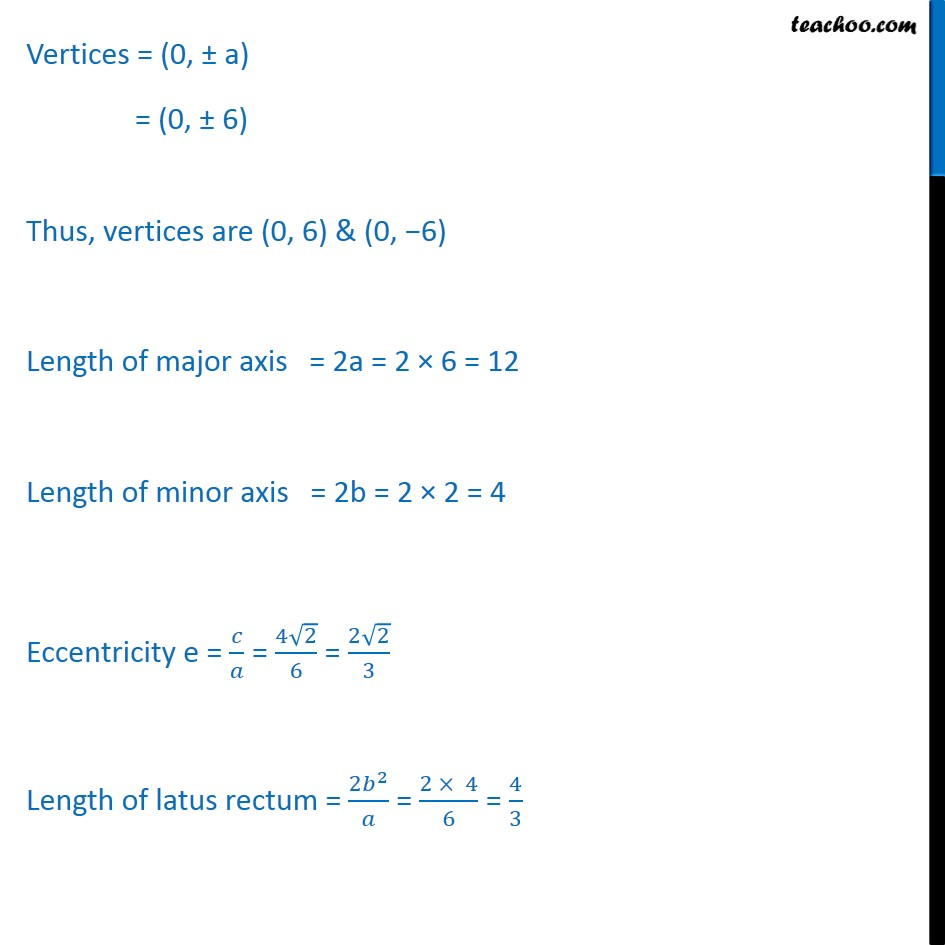Ex 10.3

Chapter 10 Class 11 Conic Sections
Serial order wiseLearn in your speed, with individual attention - Teachoo Maths 1-on-1 Class

### Transcript

Ex 10.3, 7 Find the coordinates of the foci, the vertices, the length of major axis, the minor axis, the eccentricity and the length of the latus rectum of the ellipse 36x2 + 4y2 = 144 Given 36x2 + 4y2 = 144. Dividing equation by 144 36 2 144 + 4 2 144 = 1 1 4 x2 + 1 36 y2 = 1 Since 4 < 36 Above equation is of form 2 2 + 2 2 = 1 Comparing (1) and (2) We know that c2 = a2 b2 c2 = 32 c = 32 c = 4 Coordinate of foci = (0, c) = (0, 4 2 ) So coordinates of foci are (0, 4 2 ), (0, 4 2 ) Vertices = (0, a) = (0, 6) Thus, vertices are (0, 6) & (0, 6) Length of major axis = 2a = 2 6 = 12 Length of minor axis = 2b = 2 2 = 4 Eccentricity e = = 4 2 6 = 2 2 3 Length of latus rectum = 2 2 = 2 4 6 = 4 3